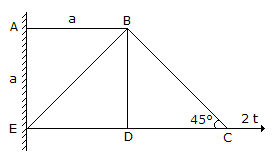# Civil Engineering - Applied Mechanics

### Exercise :: Applied Mechanics - Section 5

26.

In the structure shown in below figure, the member which carries zero force, isA. AB B. BC C. BE D. BD E. All the above.

Explanation:

No answer description available for this question. Let us discuss.

27.

When a body falls freely under gravitational force, it possesses

 A. maximum weight B. minimum weight C. no weight D. no effect on its weight.

Explanation:

No answer description available for this question. Let us discuss.

28.

A rigid body suspended Vertically at a point and oscillating with a small amplitude under the action of the force of gravity, is called

 A. simple pendulum B. compour pendulum C. Second's pendulum D. none of these.

Explanation:

No answer description available for this question. Let us discuss.

29.

If a ball which is dropped from a height of 2.25 m on a smooth floor attains the height of bounce equal to 1.00 m, the coefficient of the restitution between the ball and floor, is

 A. 0.25 B. 0.5 C. 0.67 D. 0.33 E. 0.75

Explanation:

No answer description available for this question. Let us discuss.

30.

The piston of a steam engine moves with a simple harmonic motion. The crank rotates 120 r.p.m. and the stroke length is 2 metres. The linear velocity of the piston when it is at a distance of 0.5 metre from the centre, is

 A. 5.88 m/sec B. 8.88 m/sec C. 10.88 m/sec D. 12.88 m/sec.